Mike, can you show best way to solve this insane question!? (#38 with Calculator OCT 1, 2022 SAT)

Two numbers, a and b, are each greater than zero, and 4 times the square root of a is equal to 9 times the cube root of b. If a=2/3, for what value of x is a^x equal to b?

Well, let me admit up front that I definitely didn’t see the pathway all the way to the end when I started answering this; my mental scratch paper ended up being a little bit of a mess before I could see where I was going. I say this because it’s important to expect to have to grind the hard ones out sometimes. Don’t let yourself get paralyzed if you don’t see where to go. Sometimes you gotta just play with it!

Start by translating the words into an equation.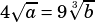I want to be free of those radicals, so let’s raise everything to the 6th power. But even though we’re in the calculator section so we don’t need to be afraid of big numbers, we shouldn’t just blindly use them. My spider sense is tingling a bit that the constants in this question are were 2, 3, 4, and 9: a couple prime numbers and their squares. AND, remember we’re on a grid-in question, so when all is said and done we’re probably going to have something nice and neat. So let’s keep everything nice and neat all the way through by working with prime factors rather than big numbers.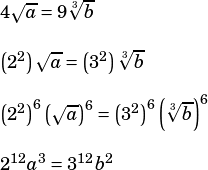Now let’s plug in the value we have for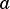, still sticking with prime factors. Then we’ll solve for.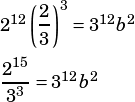Whoa, now we’re getting somewhere! Remember,. Let’s divide both sides by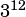.So there we go. If we know the above, and we know that, then we can substitute to get the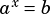form the question is looking for.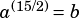The answer is.Becky says:

Mike, On #38, how did you know to raise to 6th power to lose the radicals?Mike McClenathan says:

Well, I wrote the equation with radicals because that feels like the natural translation from the words in the problem, but for me I’m always thinking about radicals as fractional exponents., and.

So in thinking about how to get rid of those fractions, what do I have to multiply them by? The least common multiple: 6.

But because those fractions are exponents, I have to remember my exponent rules. I raise the powers to a power to do that multiplication and neutralize those pesky fractions.

Does that make sense?Becky says:

It sure does! Thanks!